id.value = 3


## 语法制导定义（SDD）

SDD

• 将每个文法符号和一个语义属性集合相关联
• 将每个产生式和一组语义规则相关联，这些规则用于计算该产生式中各文法符号的属性值

$D \to TL$ L.inh = T.type
$T \to \text{int}$ T.type = int
$T \to \text{real}$ T.type = real
$L \to L_1, \text{id}$ L1.ih = L.inh

### 语法制导翻译方案（SDT）

SDT 是在产生式右部嵌入了程序片段的 CFG, 这些程序片段称为语义动作。按照惯例，语义动作放在花括号内。

### SDD 的求值顺序

$$\begin{array}{|l|l|l|} \hline & {\text { 产生式}} & {\text { 语义规则}} \\ \hline(1) & D \rightarrow T L & \text { L.in }=T \text {.type } \\ (2) & T \rightarrow \text { int } & \text { T.type }=\text { int } \\ (3) & T \rightarrow \text { real } & \text { T.type }=\text { real } \\ (4) & L \rightarrow L_{1}, \text { id } & L_{1} . \text { in }=\text { L.in } \\ & & \text { addtype(id.lexeme, } \text { L.in }) \\ (5) & L \rightarrow \text { id } & \text { addtype(id.lexeme, } \text { L.in }) \\ \hline \end{array}$$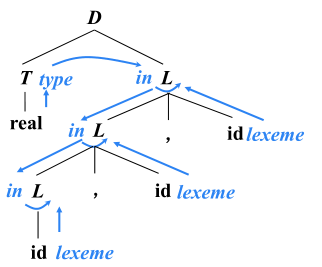S-SDD：只存在综合属性的 SDD。

L-SDD：即依赖图的边只能从左到右，不能从右到左。（产生式 $A \to X_1 X_2 \cdots X_n$ 的右部符号 $X_i$ 的属性，不能依赖于右边的 $X_j (j>i)$ 的符号的属性）这是为了禁止循环依赖。

### 语法制导翻译方案（SDT）

SDT 是 SDD 的实例。

S-SDD 到 SDT 的方法：将每个语义动作放到产生式的末尾即可。

【例子】根据下面的 SDD，为表达式 $(4 \star 7+1) \star 2$ 建立注释分析树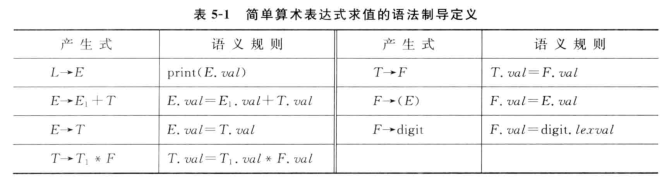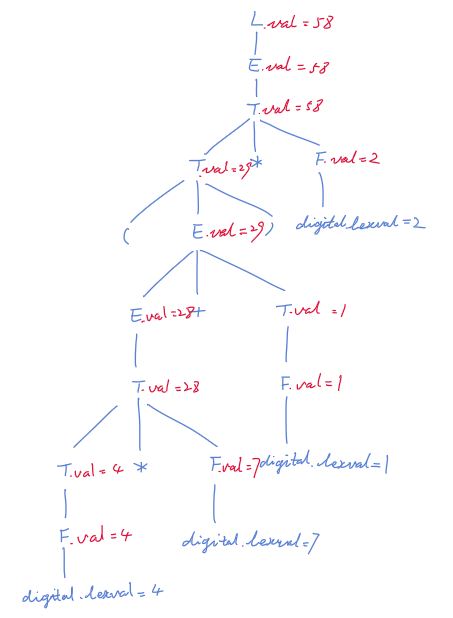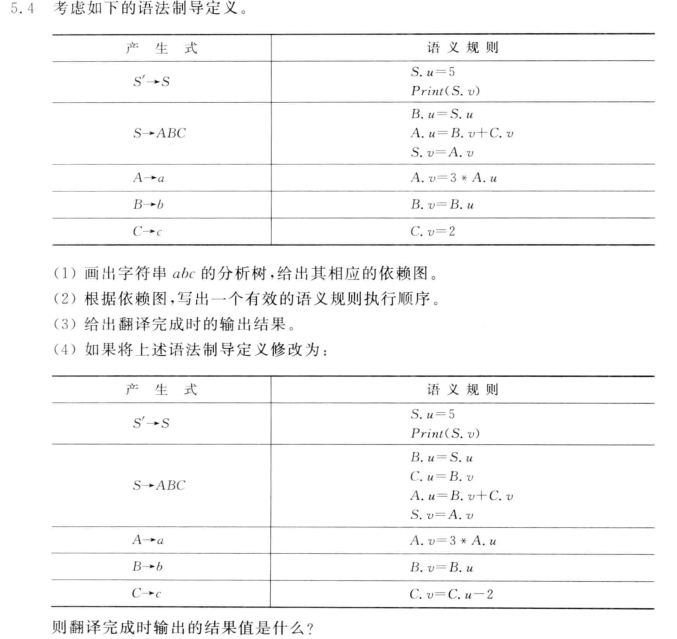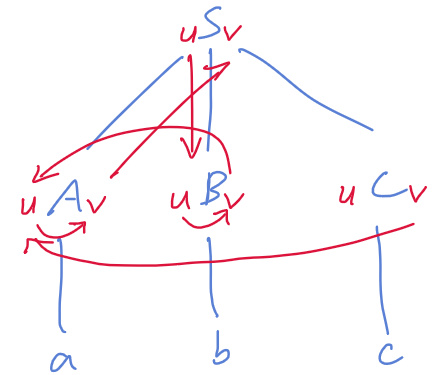（2）拓扑排序后得到：

C.v = 2
B.u = S.u		// S.u
B.v = B.u		// S.u
A.u = B.v + C.v	// S.u + 2
A.v = 3 * A.u	// 3(S.u + 2)
S.v = A.v


（3）3(S.u + 2)

（4）

B.u = S.u
B.v = B.u		// S.u
C.u = B.v		// S.u
C.v = C.u - 2	// S.u - 2
A.u = B.v + C.v // 2S.u - 2
A.v = 3*A.u		// 6(S.u-1)
S.v = A.v		// 6(S.u-1)


6(S.u-1)

1. 翻译目标：计算表达式的值
2. 确定产生式的语义
1. $e\to E_1+T$

SDD：（CFG,attrs, rules）对于每个产生式 $A \to \alpha$，都有一组关联的语义规则 $b = f (c_1, c_2, \cdots ,c_n)$

$b$ 依赖 $c_1, c_2, \cdots ,c_n$

N 上的非终结符 A 的综合属性：一个与 N 的产生式（$A \to \cdots$）关联的语义规则所定义的属性

N 上的非终结符 B 的继承属性：一个与 N 的父节点的产生式（$A \to \cdots$）关联的语义规则所定义的属性

Synthesized attribute an attribute defined wholly in terms of the attributes of the node, its children, and constants

Inherited attribute an attribute defined wholly in terms of the node’s own attributes and those of its siblings or its parent in the parse tree (plus constants)

S-attributed SDT :

• If an SDT uses only synthesized attributes, it is called as S-attributed SDT.
• S-attributed SDTs are evaluated in bottom-up parsing, as the values of the parent nodes depend upon the values of the child nodes.
• Semantic actions are placed in rightmost place of RHS.

L-attributed SDT:

• If an SDT uses both synthesized attributes and inherited attributes with a restriction that inherited attribute can inherit values from left siblings only, it is called as L-attributed SDT.
• Attributes in L-attributed SDTs are evaluated by depth-first and left-to-right parsing manner.
• Semantic actions are placed anywhere in RHS.